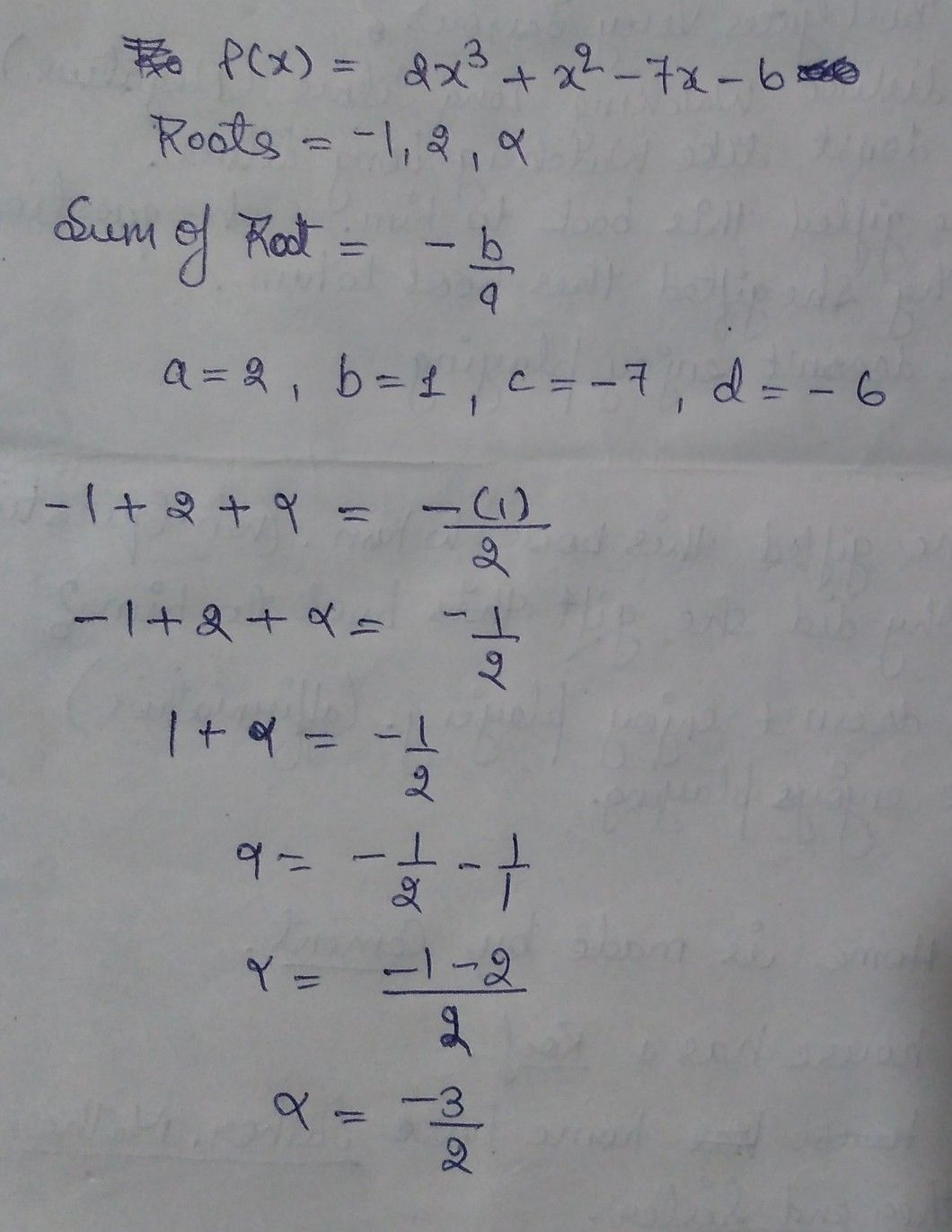Symbol
Problem$3$ Single Answer Type Review Later $t-1,2,αareihe$ $2x^{3}+x^{2}-7x$ roots $0t$ $=0,$ then $N$ is $A$ $\dfrac {3} {2}$
7th-9th grade
Other
Search count: 107
SolutionQanda teacher - Himani_